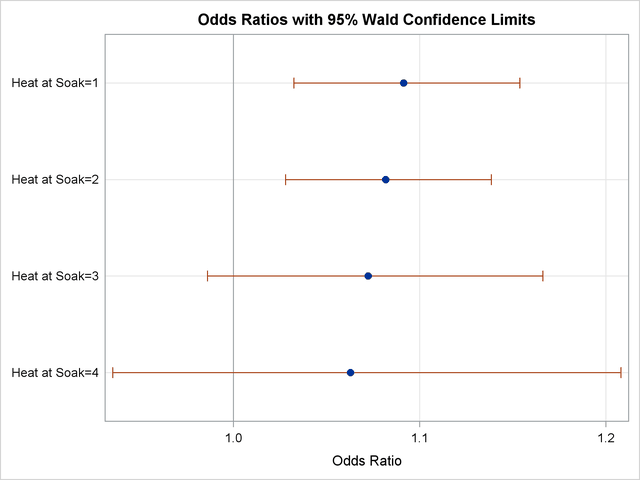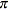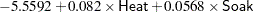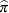# Getting Started: LOGISTIC Procedure

The LOGISTIC procedure is similar in use to the other regression procedures in the SAS System. To demonstrate the similarity, suppose the response variable y is binary or ordinal, and x1 and x2 are two explanatory variables of interest. To fit a logistic regression model, you can specify a MODEL statement similar to that used in the REG procedure. For example:

```proc logistic;
model y=x1 x2;
run;
```

The response variable y can be either character or numeric. PROC LOGISTIC enumerates the total number of response categories and orders the response levels according to the response variable option ORDER= in the MODEL statement.

You can also input binary response data that are grouped. In the following statements, n represents the number of trials and r represents the number of events:

```proc logistic;
model r/n=x1 x2;
run;
```

The following example illustrates the use of PROC LOGISTIC. The data, taken from Cox and Snell (1989, pp. 10–11), consist of the number, r, of ingots not ready for rolling, out of n tested, for a number of combinations of heating time and soaking time.

```data ingots;
input Heat Soak r n @@;
datalines;
7 1.0 0 10  14 1.0 0 31  27 1.0 1 56  51 1.0 3 13
7 1.7 0 17  14 1.7 0 43  27 1.7 4 44  51 1.7 0  1
7 2.2 0  7  14 2.2 2 33  27 2.2 0 21  51 2.2 0  1
7 2.8 0 12  14 2.8 0 31  27 2.8 1 22  51 4.0 0  1
7 4.0 0  9  14 4.0 0 19  27 4.0 1 16
;
```

The following invocation of PROC LOGISTIC fits the binary logit model to the grouped data. The continous covariates Heat and Soak are specified as predictors, and the bar notation ("|") includes their interaction, Heat*Soak. The ODDSRATIO statement produces odds ratios in the presence of interactions, and a graphical display of the requested odds ratios is produced when ODS Graphics is enabled.

```ods graphics on;
proc logistic data=ingots;
model r/n = Heat | Soak;
oddsratio Heat / at(Soak=1 2 3 4);
run;
ods graphics off;
```

The results of this analysis are shown in the following figures. PROC LOGISTIC first lists background information in Figure 53.1 about the fitting of the model. Included are the name of the input data set, the response variable(s) used, the number of observations used, and the link function used.

Figure 53.1 Binary Logit Model
The LOGISTIC Procedure

Model Information
Data Set WORK.INGOTS
Response Variable (Events) r
Response Variable (Trials) n
Model binary logit
Optimization Technique Fisher's scoring

 Number of Observations Read 19 19 387 387

The "Response Profile" table (Figure 53.2) lists the response categories (which are Event and Nonevent when grouped data are input), their ordered values, and their total frequencies for the given data.

Figure 53.2 Response Profile with Events/Trials Syntax
Response Profile
Ordered
Value
Binary Outcome Total
Frequency
1 Event 12
2 Nonevent 375

Model Convergence Status
Convergence criterion (GCONV=1E-8) satisfied.

The "Model Fit Statistics" table (Figure 53.3) contains Akaike’s information criterion (AIC), the Schwarz criterion (SC), and the negative of twice the log likelihood (–2 Log L) for the intercept-only model and the fitted model. AIC and SC can be used to compare different models, and the ones with smaller values are preferred. Results of the likelihood ratio test and the efficient score test for testing the joint significance of the explanatory variables (Soak, Heat, and their interaction) are included in the "Testing Global Null Hypothesis: BETA=0" table (Figure 53.3); the small p-values reject the hypothesis that all slope parameters are equal to zero.

Figure 53.3 Fit Statistics and Hypothesis Tests
Model Fit Statistics
Criterion Intercept
Only
Intercept
and
Covariates
With
Constant
AIC 108.988 103.222 35.957
SC 112.947 119.056 51.791
-2 Log L 106.988 95.222 27.957

Testing Global Null Hypothesis: BETA=0
Test Chi-Square DF Pr > ChiSq
Likelihood Ratio 11.7663 3 0.0082
Score 16.5417 3 0.0009
Wald 13.4588 3 0.0037

The "Analysis of Maximum Likelihood Estimates" table in Figure 53.4 lists the parameter estimates, their standard errors, and the results of the Wald test for individual parameters. Note that the Heat*Soak parameter is not significantly different from zero (p=0.727), nor is the Soak variable (p=0.6916).

Figure 53.4 Parameter Estimates
Analysis of Maximum Likelihood Estimates
Parameter DF Estimate Standard
Error
Wald
Chi-Square
Pr > ChiSq
Intercept 1 -5.9901 1.6666 12.9182 0.0003
Heat 1 0.0963 0.0471 4.1895 0.0407
Soak 1 0.2996 0.7551 0.1574 0.6916
Heat*Soak 1 -0.00884 0.0253 0.1219 0.7270

The "Association of Predicted Probabilities and Observed Responses" table (Figure 53.5) contains four measures of association for assessing the predictive ability of a model. They are based on the number of pairs of observations with different response values, the number of concordant pairs, and the number of discordant pairs, which are also displayed. Formulas for these statistics are given in the section Rank Correlation of Observed Responses and Predicted Probabilities.

Figure 53.5 Association Table
Association of Predicted Probabilities and
Observed Responses
Percent Concordant 70.9 Somers' D 0.537
Percent Discordant 17.3 Gamma 0.608
Percent Tied 11.8 Tau-a 0.032
Pairs 4500 c 0.768

The ODDSRATIO statement produces the "Odds Ratio Estimates and Wald Confidence Intervals" table (Figure 53.6), and a graphical display of these estimates is shown in Figure 53.7. The differences between the odds ratios are small compared to the variability shown by their confidence intervals, which confirms the previous conclusion that the Heat*Soak parameter is not significantly different from zero.

Figure 53.6 Odds Ratios of Heat at Several Values of Soak
Odds Ratio Estimates and Wald Confidence Intervals
Label Estimate 95% Confidence Limits
Heat at Soak=1 1.091 1.032 1.154
Heat at Soak=2 1.082 1.028 1.139
Heat at Soak=3 1.072 0.986 1.166
Heat at Soak=4 1.063 0.935 1.208

Figure 53.7 Plot of Odds Ratios of Heat at Several Values of SoakSince the Heat*Soak interaction is nonsignificant, the following statements fit a main-effects model:

```proc logistic data=ingots;
model r/n = Heat Soak;
run;
```

The results of this analysis are shown in the following figures. The model information and response profiles are the same as those in Figure 53.1 and Figure 53.2 for the saturated model. The "Model Fit Statistics" table in Figure 53.8 shows that the AIC and SC for the main-effects model are smaller than for the saturated model, indicating that the main-effects model might be the preferred model. As in the preceding model, the "Testing Global Null Hypothesis: BETA=0" table indicates that the parameters are significantly different from zero.

Figure 53.8 Fit Statistics and Hypothesis Tests
The LOGISTIC Procedure

Model Fit Statistics
Criterion Intercept
Only
Intercept
and
Covariates
With
Constant
AIC 108.988 101.346 34.080
SC 112.947 113.221 45.956
-2 Log L 106.988 95.346 28.080

Testing Global Null Hypothesis: BETA=0
Test Chi-Square DF Pr > ChiSq
Likelihood Ratio 11.6428 2 0.0030
Score 15.1091 2 0.0005
Wald 13.0315 2 0.0015

The "Analysis of Maximum Likelihood Estimates" table in Figure 53.9 again shows that the Soak parameter is not significantly different from zero (p=0.8639). The odds ratio for each effect parameter, estimated by exponentiating the corresponding parameter estimate, is shown in the "Odds Ratios Estimates" table (Figure 53.9), along with 95% Wald confidence intervals. The confidence interval for the Soak parameter contains the value 1, which also indicates that this effect is not significant.

Figure 53.9 Parameter Estimates and Odds Ratios
Analysis of Maximum Likelihood Estimates
Parameter DF Estimate Standard
Error
Wald
Chi-Square
Pr > ChiSq
Intercept 1 -5.5592 1.1197 24.6503 <.0001
Heat 1 0.0820 0.0237 11.9454 0.0005
Soak 1 0.0568 0.3312 0.0294 0.8639

Odds Ratio Estimates
Effect Point Estimate 95% Wald
Confidence Limits
Heat 1.085 1.036 1.137
Soak 1.058 0.553 2.026

Association of Predicted Probabilities and
Observed Responses
Percent Concordant 64.4 Somers' D 0.460
Percent Discordant 18.4 Gamma 0.555
Percent Tied 17.2 Tau-a 0.028
Pairs 4500 c 0.730

Using these parameter estimates, you can calculate the estimated logit ofasFor example, if Heat7 and Soak1, then logit. Using this logit estimate, you can calculateas follows:This gives the predicted probability of the event (ingot not ready for rolling) for Heat7 and Soak1. Note that PROC LOGISTIC can calculate these statistics for you; use the OUTPUT statement with the PREDICTED= option, or use the SCORE statement.

To illustrate the use of an alternative form of input data, the following program creates the ingots data set with the new variables NotReady and Freq instead of n and r. The variable NotReady represents the response of individual units; it has a value of 1 for units not ready for rolling (event) and a value of 0 for units ready for rolling (nonevent). The variable Freq represents the frequency of occurrence of each combination of Heat, Soak, and NotReady. Note that, compared to the previous data set, NotReady1 implies Freqr, and NotReady0 implies Freqnr.

```data ingots;
input Heat Soak NotReady Freq @@;
datalines;
7 1.0 0 10  14 1.0 0 31  14 4.0 0 19  27 2.2 0 21  51 1.0 1  3
7 1.7 0 17  14 1.7 0 43  27 1.0 1  1  27 2.8 1  1  51 1.0 0 10
7 2.2 0  7  14 2.2 1  2  27 1.0 0 55  27 2.8 0 21  51 1.7 0  1
7 2.8 0 12  14 2.2 0 31  27 1.7 1  4  27 4.0 1  1  51 2.2 0  1
7 4.0 0  9  14 2.8 0 31  27 1.7 0 40  27 4.0 0 15  51 4.0 0  1
;
```

The following statements invoke PROC LOGISTIC to fit the main-effects model by using the alternative form of the input data set:

```proc logistic data=ingots;
freq Freq;
run;
```

Results of this analysis are the same as the preceding single-trial main-effects analysis. The displayed output for the two runs are identical except for the background information of the model fit and the "Response Profile" table shown in Figure 53.10.

Figure 53.10 Response Profile with Single-Trial Syntax
The LOGISTIC Procedure

Response Profile
Ordered
Value